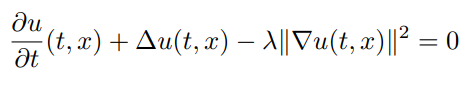# Solving a 100-dimensional Hamilton-Jacobi-Bellman Equation

First, here's a fully working code for the solution of a 100-dimensional Hamilton-Jacobi-Bellman equation that takes a few minutes on a laptop:

using NeuralPDE
using Flux
using DifferentialEquations
using LinearAlgebra
d = 100 # number of dimensions
X0 = fill(0.0f0, d) # initial value of stochastic control process
tspan = (0.0f0, 1.0f0)
λ = 1.0f0

g(X) = log(0.5f0 + 0.5f0 * sum(X.^2))
f(X,u,σᵀ∇u,p,t) = -λ * sum(σᵀ∇u.^2)
μ_f(X,p,t) = zero(X)  # Vector d x 1 λ
σ_f(X,p,t) = Diagonal(sqrt(2.0f0) * ones(Float32, d)) # Matrix d x d
prob = TerminalPDEProblem(g, f, μ_f, σ_f, X0, tspan)
hls = 10 + d # hidden layer size
# sub-neural network approximating solutions at the desired point
u0 = Flux.Chain(Dense(d, hls, relu),
Dense(hls, hls, relu),
Dense(hls, 1))
# sub-neural network approximating the spatial gradients at time point
σᵀ∇u = Flux.Chain(Dense(d + 1, hls, relu),
Dense(hls, hls, relu),
Dense(hls, hls, relu),
Dense(hls, d))
pdealg = NNPDENS(u0, σᵀ∇u, opt=opt)
@time ans = solve(prob, pdealg, verbose=true, maxiters=100, trajectories=100,
alg=EM(), dt=1.2, pabstol=1f-2)

Now, let's explain the details!

## H-J-B equation

The Hamilton-Jacobi-Bellman equation is the solution to a stochastic optimal control problem.

### Symbolic Solution

Here, we choose to solve the classical Linear Quadratic Gaussian (LQG) control problem of 100 dimensions, which is governed by the SDE dX_t = 2sqrt(λ)c_t dt + sqrt(2)dW_t where c_t is a control process. The solution to the optimal control is given by a PDE of the form:with terminating condition g(X) = log(0.5f0 + 0.5f0*sum(X.^2)).

## Solving LQG Problem with Neural Net

### Define the Problem

To get the solution above using the TerminalPDEProblem, we write:

d = 100 # number of dimensions
X0 = fill(0.0f0,d) # initial value of stochastic control process
tspan = (0.0f0, 1.0f0)
λ = 1.0f0

g(X) = log(0.5f0 + 0.5f0*sum(X.^2))
f(X,u,σᵀ∇u,p,t) = -λ*sum(σᵀ∇u.^2)
μ_f(X,p,t) = zero(X)  #Vector d x 1 λ
σ_f(X,p,t) = Diagonal(sqrt(2.0f0)*ones(Float32,d)) #Matrix d x d
prob = TerminalPDEProblem(g, f, μ_f, σ_f, X0, tspan)

### Define the Solver Algorithm

As described in the API docs, we now need to define our NNPDENS algorithm by giving it the Flux.jl chains we want it to use for the neural networks. u0 needs to be a d dimensional -> 1 dimensional chain, while σᵀ∇u needs to be d+1 dimensional to d dimensions. Thus we define the following:

hls = 10 + d #hidden layer size
#sub-neural network approximating solutions at the desired point
u0 = Flux.Chain(Dense(d,hls,relu),
Dense(hls,hls,relu),
Dense(hls,1))
# sub-neural network approximating the spatial gradients at time point
σᵀ∇u = Flux.Chain(Dense(d+1,hls,relu),
Dense(hls,hls,relu),
Dense(hls,hls,relu),
Dense(hls,d))
pdealg = NNPDENS(u0, σᵀ∇u, opt=opt)

### Solving with Neural Net

@time ans = solve(prob, pdealg, verbose=true, maxiters=100, trajectories=100,
alg=EM(), dt=0.2, pabstol = 1f-2)


Here we want to solve the underlying neural SDE using the Euler-Maruyama SDE solver with our chosen dt=0.2, do at most 100 iterations of the optimizer, 100 SDE solves per loss evaluation (for averaging), and stop if the loss ever goes below 1f-2.